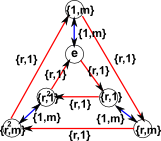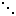# Maths - Dihedral Groups - Datasheet

## Cayley Table

The Cayley table has four quadrants shown here in red,green,blue and orange, each of these quadrants is similar to the Cayley table for a cyclic group:

 {1,1} {r,1} {r²,1} {1,m} {r,m} {r²,m} {r,1} {r²,1} {1,1} {r,m} {r²,m} {1,m} {r²,1} {1,1} {r,1} {r²,m} {1,m} {r,m} {1,m} {r,m} {r²,m} {1,1} {r,1} {r²,1} {r,m} {r²,m} {1,m} {r,1} {r²,1} {1,1} {r²,m} {1,m} {r,m} {r²,1} {1,1} {r,1}

## Cayley Graph

There are two cycles that go in opposite directions## Cyclic Notation

The two generators are:

1. A complete cycle
2. A set of 2-element cycles which swap opposite points

(1,2,3..n) , ( 1 n ) ( 2 (n-1)… )

## Group Presentation

Thereare two generators, r (for rotate) is like a cyclic group, and m (for mirror) which flips the shape over.

<r , m | rn =1,r² = 1, m r m= r-1>

or equivalently:

<x , y | x² = y² = xyn = 1>

## Group Representation

One generator is a rotation of the identity matrix through 90° (the diagonal from bottom-left to top-right), the other is the nth root of the identity matix (such that lesser roots are not identity). See this page for information about taking roots of a matrix. One a matrix which will do this is an n×n matrix on the right:

 0 0 … 0 0 1 1 0 … 0 0 0 0 1 … 0 0 00 0 … 1 0 0 0 0 … 0 1 0
,
 0 0 0 0 1 0 0 0 1 0 0 0 1 0 0 0 1 0 0 0 1 0 0 0 0

## Related datasheetsSymmetry and the Monster - This is a popular science type book which traces the history leading up to the discovery of the largest symmetry groups.